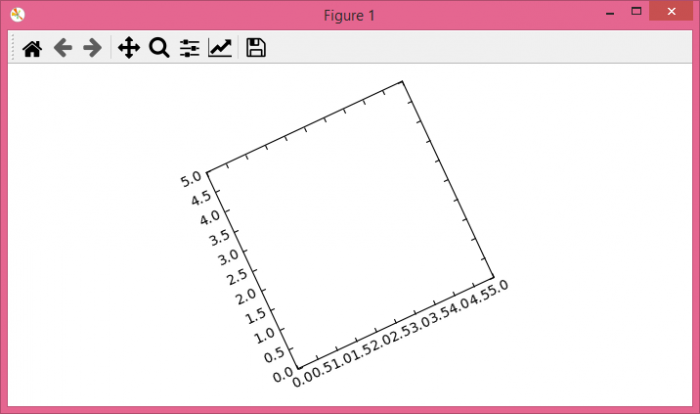# How to rotate a simple matplotlib Axes?

To rotate a simple matplotlib axes, we can take the following steps −

• Import the required packages −
import matplotlib.pyplot as plt
from matplotlib.transforms import Affine2D
import mpl_toolkits.axisartist.floating_axes as floating_axes
• Set the figure size and adjust the padding between and around the subplots.
• Create a new figure or activate an existing figure.
• Make a tuple of axes extremes.
• Add a mutable 2D affine transformation, "t". Add a rotation (in degrees) to this transform in place.
• Add a transform from the source (curved) coordinate to target (rectilinear) coordinate.
• Add a floating axes "h" with the current figure with GridHelperCurveLinear() instance.
• Add an 'ax' to the figure as part of a subplot arrangement.
• To display the figure, use show() method.

## Example

# import the packages
import matplotlib.pyplot as plt
from matplotlib.transforms import Affine2D
import mpl_toolkits.axisartist.floating_axes as floating_axes

# set the figure size
plt.rcParams["figure.figsize"] = [7.00, 3.50]
plt.rcParams["figure.autolayout"] = True

# plot the figure
fig = plt.figure()

scales = (0, 5, 0, 5)

t = Affine2D().rotate_deg(25)

h = floating_axes.GridHelperCurveLinear(t, scales)
ax = floating_axes.FloatingSubplot(fig, 111, grid_helper=h)

plt.show()